# Balancing Chemical Equations pp 125 133 Chemical reactions

• Slides: 14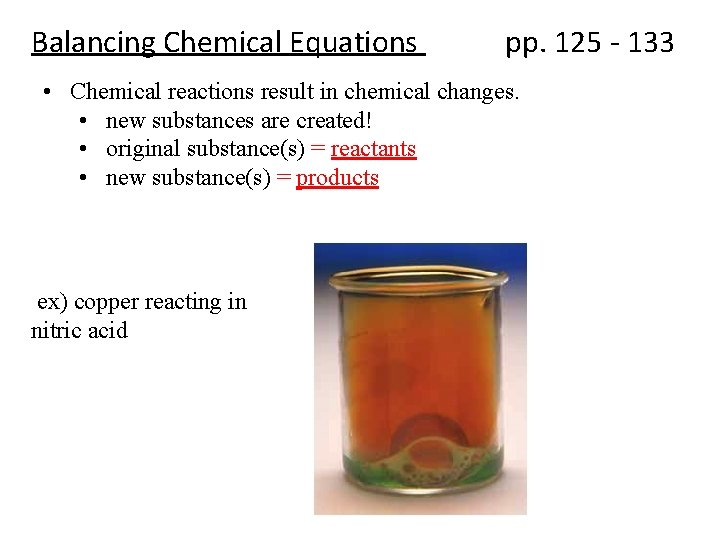Balancing Chemical Equations pp. 125 - 133 • Chemical reactions result in chemical changes. • new substances are created! • original substance(s) = reactants • new substance(s) = products ex) copper reacting in nitric acid• Chemical reactions can be written in different ways. – A word equation: Nitrogen monoxide + oxygen nitrogen dioxide – A symbolic equation: 2 NO(g) + O 2(g) 2 NO 2(g) State of matter (aq) =aqueous/ dissolved in water Coefficients (s) = solid - indicate the ratio of compounds in the reaction (l) = liquid - here, there is twice as much NO and NO 2 than there is O 2 (g) = gas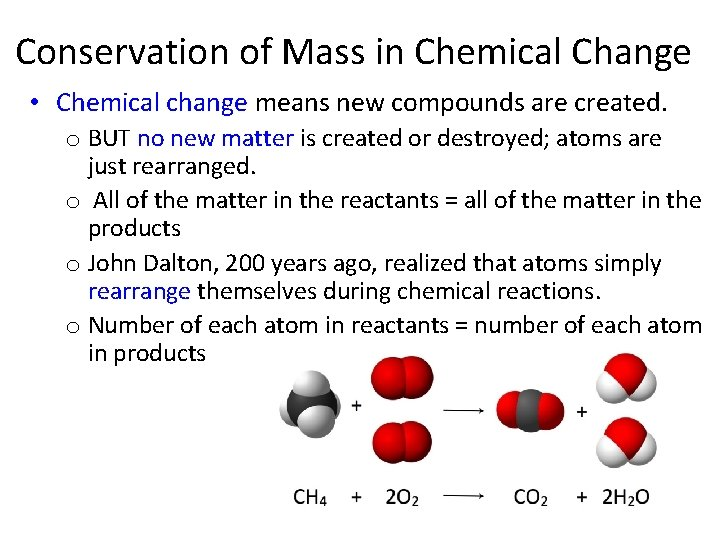Conservation of Mass in Chemical Change • Chemical change means new compounds are created. o BUT no new matter is created or destroyed; atoms are just rearranged. o All of the matter in the reactants = all of the matter in the products o John Dalton, 200 years ago, realized that atoms simply rearrange themselves during chemical reactions. o Number of each atom in reactants = number of each atom in productsThe Law of Conservation of Mass § In chemical reactions, atoms are neither created nor destroyed § Developed by Antoine Lavoisier and his wife Marie-Anne in the 1700 s § Mass of reactants = Mass of products If you could collect and measure all of the exhaust from this car, you would find that mass of reactants (gas + O 2) = mass of products (exhaust)!Writing and Balancing Chemical Equations • The simplest form of chemical equation is a word equation Potassium metal + oxygen gas potassium oxide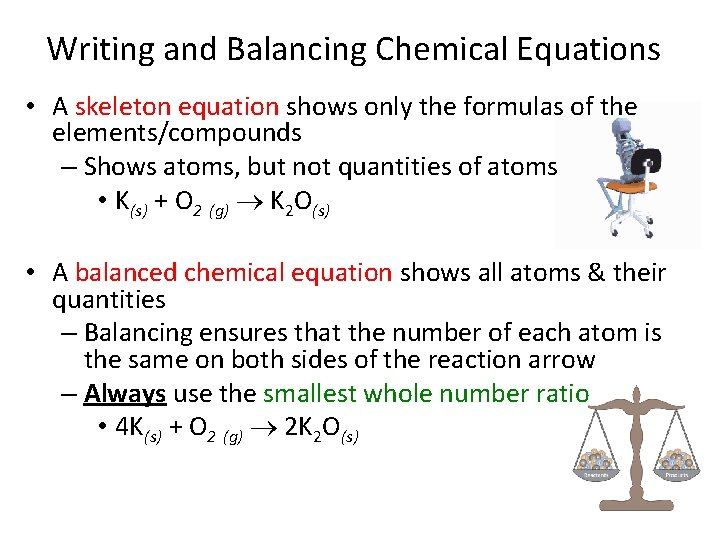Writing and Balancing Chemical Equations • A skeleton equation shows only the formulas of the elements/compounds – Shows atoms, but not quantities of atoms • K(s) + O 2 (g) K 2 O(s) • A balanced chemical equation shows all atoms & their quantities – Balancing ensures that the number of each atom is the same on both sides of the reaction arrow – Always use the smallest whole number ratio • 4 K(s) + O 2 (g) 2 K 2 O(s)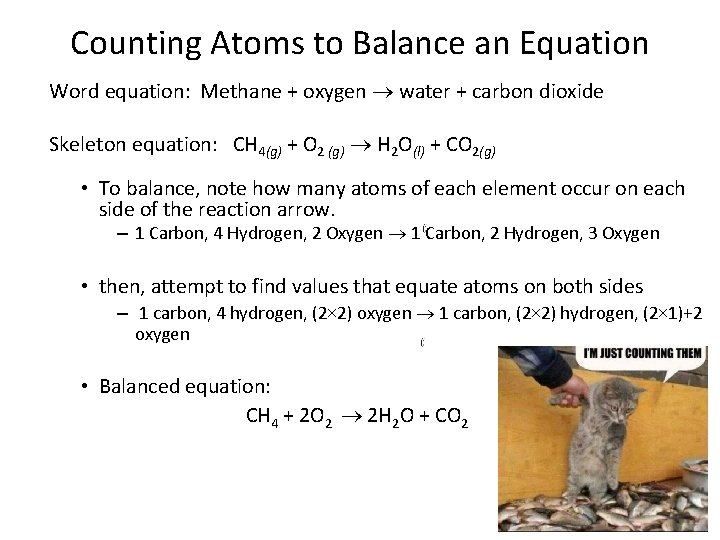Counting Atoms to Balance an Equation Word equation: Methane + oxygen water + carbon dioxide Skeleton equation: CH 4(g) + O 2 (g) H 2 O(l) + CO 2(g) • To balance, note how many atoms of each element occur on each side of the reaction arrow. – 1 Carbon, 4 Hydrogen, 2 Oxygen 1 Carbon, 2 Hydrogen, 3 Oxygen • then, attempt to find values that equate atoms on both sides – 1 carbon, 4 hydrogen, (2× 2) oxygen 1 carbon, (2× 2) hydrogen, (2× 1)+2 oxygen • Balanced equation: CH 4 + 2 O 2 2 H 2 O + CO 2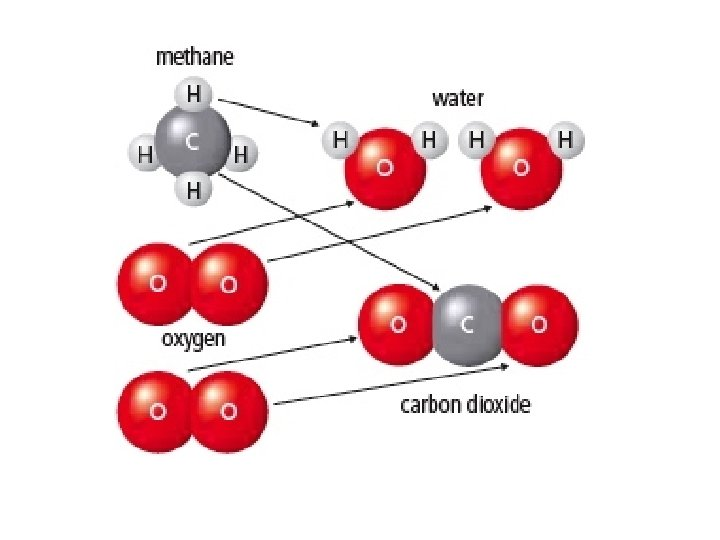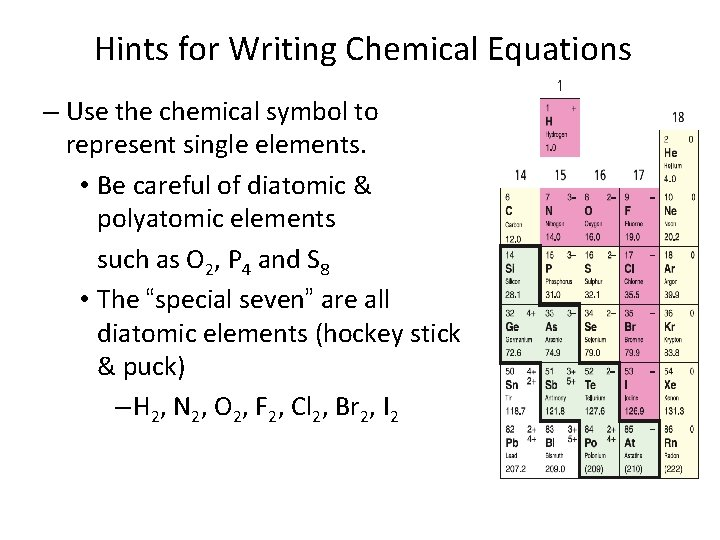Hints for Writing Chemical Equations – Use the chemical symbol to represent single elements. • Be careful of diatomic & polyatomic elements such as O 2, P 4 and S 8 • The “special seven” are all diatomic elements (hockey stick & puck) – H 2, N 2, O 2, F 2, Cl 2, Br 2, I 2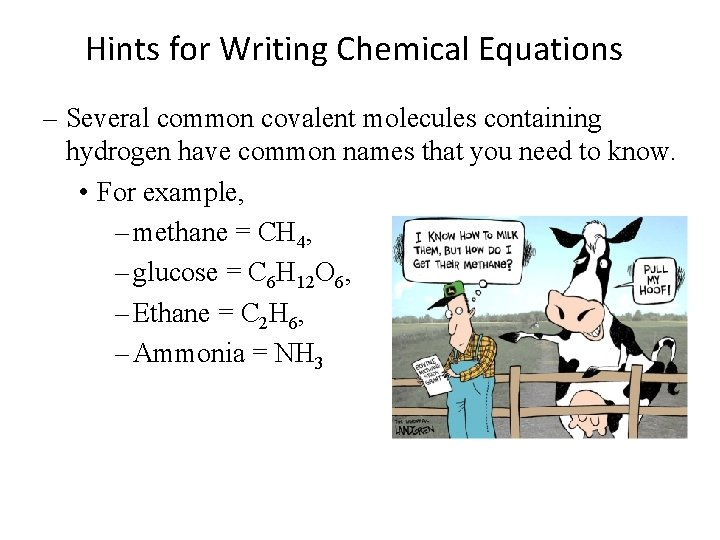Hints for Writing Chemical Equations – Several common covalent molecules containing hydrogen have common names that you need to know. • For example, – methane = CH 4, – glucose = C 6 H 12 O 6, – Ethane = C 2 H 6, – Ammonia = NH 3Strategies for Balancing Equations • Tips to balance chemical equations: – Trial and error will work, but can be very inefficient – Balance compounds first, elements last – Balance one compound at a time – Only add coefficients; NEVER change subscripts! – Balance metals first, then nonmetals, then H & O – If H and O appear in more than one place, attempt to balance them LAST – Polyatomic ions (such as SO 42–) can often be balanced as a whole group – Always double-check after you think you are finished!Balancing Equations Examples • Balance the following: 1. 2. Balance Fe: Balance Br: 3. Br = Balance Fe again: Left 1 2 x 3 6 x 2 2 Fe + Br 2 Right 1 3 x 2 6 x 2 2 Answer: 2 Fe + 3 Br 2 2 Fe. Br 3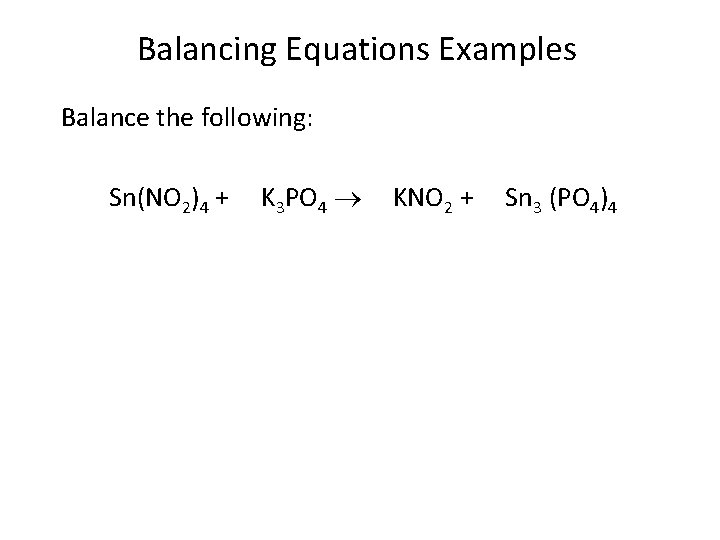Balancing Equations Examples Balance the following: Sn(NO 2)4 + K 3 PO 4 KNO 2 + Sn 3 (PO 4)4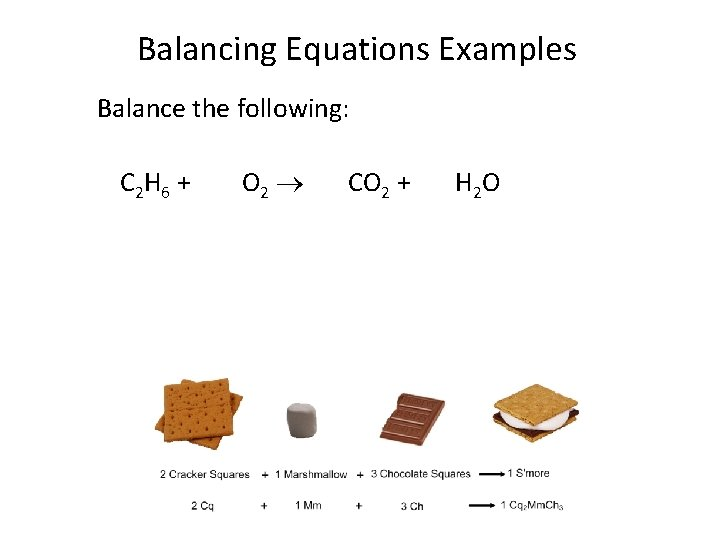Balancing Equations Examples Balance the following: C 2 H 6 + O 2 CO 2 + H 2 O